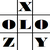anything in Excel...

It's Excel-o-lozy...

### "Calculate Cells" rather than whole Sheet or Workbook for Manual Calculation option.

www.Excelolozy.com - "CALCULATE CELLS"... this option is a dream for heavy Excel files users who set the Calculation to Manual.

Calculating the WORKBOOK (F9) or just calculating the current WORKSHEET takes loads of time.

Solution to it is “Calculate Cells”.

It just recalculates the selected range of cells rather than whole worksheet or workbook.

All that required is as follows: